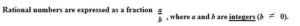#Difference Between Similar Terms and Objects

# Difference Between Rational and Irrational Numbers

The term “numbers” brings to our mind what are generally classified as positive integer values greater than zero. Other classes of numbers include whole numbers and fractions, complex and real numbers and also negative integer values.

Extending the classifications of numbers further, we encounter rational and irrational numbers. A rational number is a number that can be written as a fraction. In other words, the rational number can be written as a ratio of two numbers.Consider, for example, the number 6. It can be written as the ratio of two numbers viz. 6 and 1, leading to the ratio 6/1. Likewise, 2/3, which is written as a fraction, is a rational number.

We can, thus, define a rational number, as a number written in the form of a fraction, wherein both the numerator (the number on top) and the denominator (the number on the bottom) are whole numbers. By definition, therefore, every whole number is also a rational number.

A ratio of two large numbers such as (129,367,871)/(547,724,863) would also constitute an example of a rational number for the simple reason that both the numerator and the denominator are whole numbers.

Conversely, any number that cannot be expressed in the form of a fraction or a ratio is termed as irrational. The most commonly cited example of an irrational number is 2 (1.414213…). Another popular example of an irrational number is the numerical constant π (3.141592…).

An irrational number can be written as a decimal, but not as a fraction. Irrational numbers are not often used in daily life although they do exist on the number line. There are an infinite number of irrational numbers between 0 and 1 on the number line. An irrational number has endless non-repeating digits to the right of the decimal point.

Note that the oft-cited value of 22/7 for the constant π is in fact only one the values of π. By definition, the circumference of a circle divided by twice its radius is the value of π. This leads to multiple values of π, including, but not limited to, 333/106, 355/113 and so on1.

Only the square roots of the square numbers; i.e., the square roots of the perfect squares are rational.

√1= 1 (Rational)

√2 (Irrational)

√3 (Irrational)

√4 = 2 (Rational)

√5, √6, √7, √8 (Irrational)

√9 = 3 (Rational) and so on.

Further, we note that, only the nth roots of nth powers are rational. Thus, the 6th root of 64 is rational, because 64 is a 6th power, namely the 6th power of 2. But the 6th root of 63 is irrational. 63 is not a perfect 6th power.

Inevitably, the decimal representation of irrationals comes into picture and poses some interesting results.

When we express a rational number as a decimal, then either the decimal will be exact (as in 1/5= 0.20) or it will be inexact (as in, 1/3 ≈ 0.3333). In either case, there will be a predictable pattern of digits.  Note that when an irrational number is expressed as a decimal, then clearly it will inexact, because otherwise, the number would be rational.

Moreover, there will not be a predictable pattern of digits.  For example,

√2 ≈1.4142135623730950488016887242097

Now, with rational numbers, we occasionally encounter 1/11 = 0.0909090.

The use of both the equal sign (=) and three dots (ellipsis) implies that though is it not possible to express 1/11 exactly as a decimal, we can still approximate it with as many decimal digits as permitted to get close to 1/11.

Thus, the decimal form of 1/11 is deemed inexact. By the same token, the decimal form of  ¼ which is 0.25, is exact.

Coming to the decimal form for irrational numbers, they are going to be always inexact. Continuing with the example of 2, when we write √2 = 1.41421356237. . . (note the use of ellipsis), it immediately implies that no decimal for √2 will be exact. Further, there will not be a predictable pattern of digits. Using concepts from numerical methods, again, we can rationally approximate for as many decimal digits as till such point that we are close to √2.

Any note on rational and irrational numbers cannot end without the obligatory proof as to why √2 is irrational. In doing so, we also elucidate, the classic example of a proof by contradiction.

Suppose √2 is rational. This leads us to represent it as a ratio of two integers, say p and q.

√2 = p/q

Needless to say, p and q have no common factors, for if there were to be any common factors, we would have cancelled them out from the numerator and the denominator.

Squaring both sides of the equation, we end up with,

2 = p2 / q2

This can be conveniently written as,

p2 = 2q2

The last equation suggests that p2 is even. This is possible only if p itself is even. This in turn implies that p2 is divisible by 4. Hence, q2 and consequently q must be even. So p and q are both even which is a contradiction to our initial assumption that they have no common factors. Thus, √2 cannot be rational. Q.E.D.

### Search DifferenceBetween.net :

Custom Search

Help us improve. Rate this post!(1 votes, average: 5.00 out of 5)Loading...Email This Post : If you like this article or our site. Please spread the word. Share it with your friends/family.